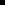### Derived Points

 Overview Guidelines Example

By default, when a derived point expression uses a device point, it uses the raw (unconverted) value of the device point.

When a derived point value is calculated:

1. Constants are assumed to be in the base units for the point.

2. The base units for all points in the expression are used.

3. The result is then converted to the current active measurement system units for the derived point.

Assign a base Unit ID for a derived point that is consistent with the base Unit IDs of the points in its expression and use constants that are consistent with the base Unit ID for the derived point.

If you are using Measurement Units and want your derived points to be calculated correctly, you need to use the Engineering Units (EU) value for device points in your derived point expressions. You can do this in one of two ways:Set the PTMDP_DO_EU_CONV global parameter to one (1) to automatically use the EU value of device points in all derived point expressions.Use the EU_CONV function for every device point you use in a derived point expression to convert the device points to their EU values.

SOURCE is a device point with a Base Unit ID of inches.

DEST is a derived point with an expression: SOURCE+10.0 (inches).

The value of SOURCE is 1 inch.

You will use Measurement Units conversion to convert the project from inches to centimeters.

Don'tAssign DEST a measurement unit of centimeters in the Point Properties box Conversion tab, which is different from SOURCE's assigned measurement unit, inches.Leave DEST's measurement unit (in the Conversion tab) blank.

In either case you are creating an inconsistent condition which may produce confusing results after conversion.

Do

Assign DEST a base measurement unit of inches in the Point Properties box Conversion tab.

The expression, SOURCE+10.0, will be calculated as 1 inch +10 (inches) = 11 inches

Because DEST has a base measurement unit of inches, it will be included in the Measurement Unit conversion and will display as 27.94 centimeters.

 Some guidelines for measurement units.